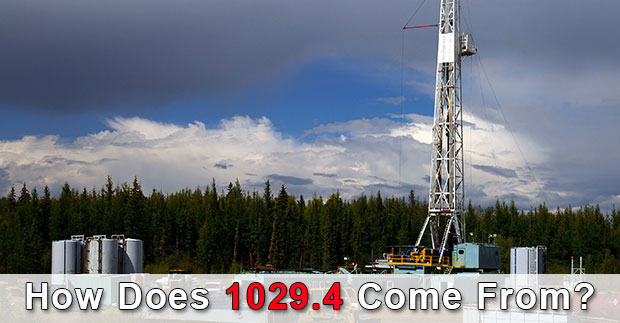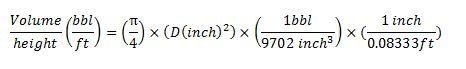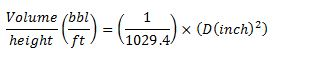# How Does 1029.4 Come From?

1029.4 is used in several calculations in the oilfield and we’ve been asked about what is 1029.4, how it comes from, why it needs to be this figure so in this article, we will show you how 1029.4 comes from.First of all, we would like to give someone about the background of this figure. The 1029.4 is widely used for capacity calculations. The following equations utilizing 1029.4 are listed below;

Annular capacity, bbl/ft = (OD2 – ID2) ÷ 1029.4

Internal capacity, bbl/ft = ID2 ÷ 1029.4

Where;

ID, OD are in inch.

Annular capacity and internal capacity are in bbl/ft.

As you can see, 1029.4 is the unit conversion used to convert square inch into bbl/ft.

Let’s see how we can find this figure mathematically.

Area of circle (square inch) = (π÷4) x D2

D is diameter in inch.

For oilfield unit, the diameter (D) is inch.

In term of mathematic, Area (square inch) is equal to Volume per Height, cubic inch (in3) per inch (in).

Cubic inch per inch is not typically used in oilfield and oilfield unit usually uses bbl for volume and ft for length. Therefore, we need to convert from in3/in to bbl/ft.

The following figures are unit conversions used for the calculation.

1 bbl = 9702 cubic inch (in3)

1 inch = 0.08333 ft

Based on the unit conversions and Area of circle formula, we can put everything together like this.This is the final formula.You can see that 1029.4 is the final conversion unit for this formula.

Share the joy